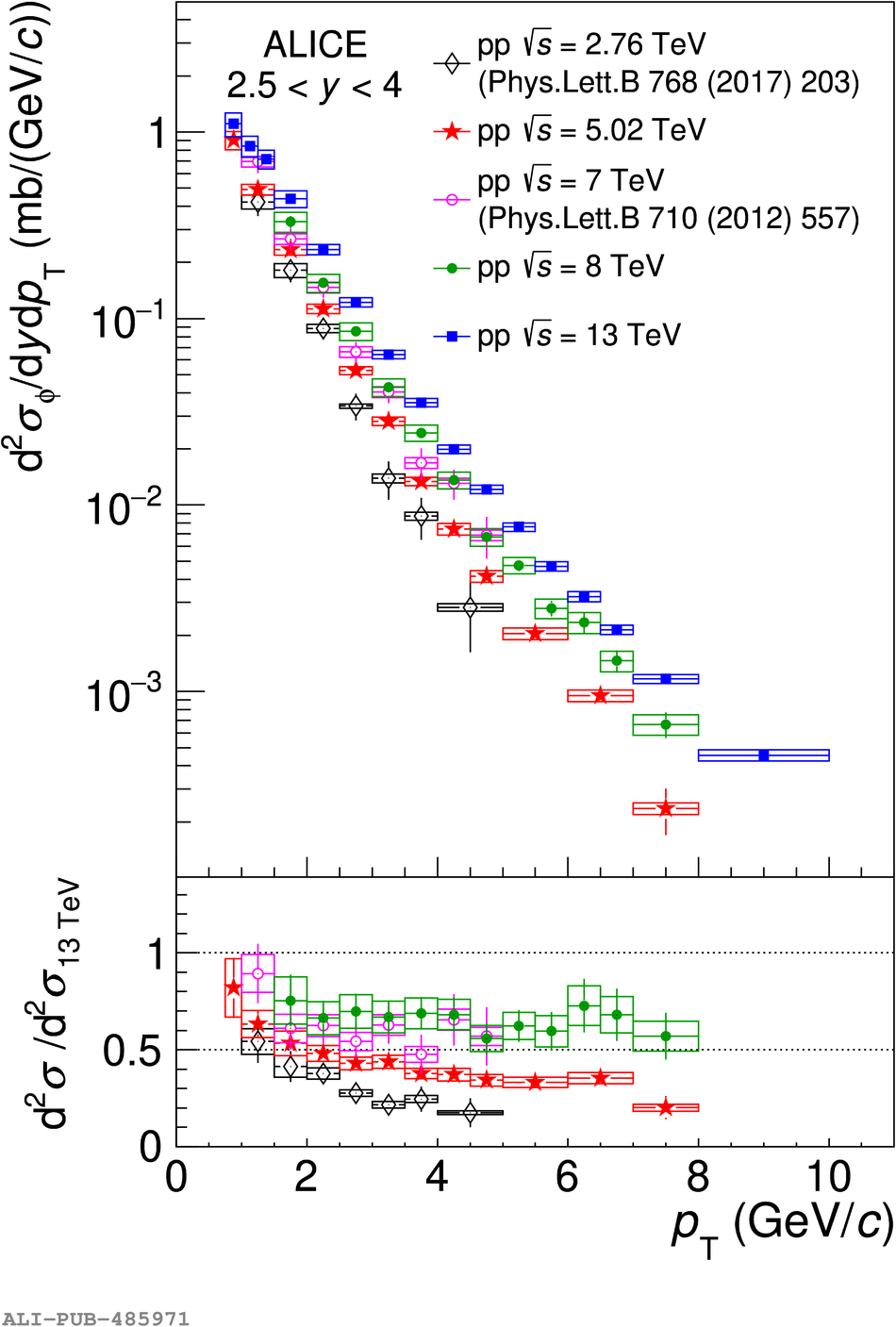Figure 3

 Top: differential $\phi$ meson production cross section $\mathrm{d}^2\sigma/(\mathrm{d}y\mathrm{d}\pt$) as a function of $\pt$ at $\sqrt{s}=$2.76, 5.02, 7, 8 and 13~TeV The boxes represent the systematic uncertainties, the error bars the statistical uncertainties. Bottom: ratio between the cross sections measured at several energies to the one obtained at $\sqrt{s}=13$~TeV.